Ex 10.2

Chapter 10 Class 11 Straight Lines (Term 1)
Serial order wise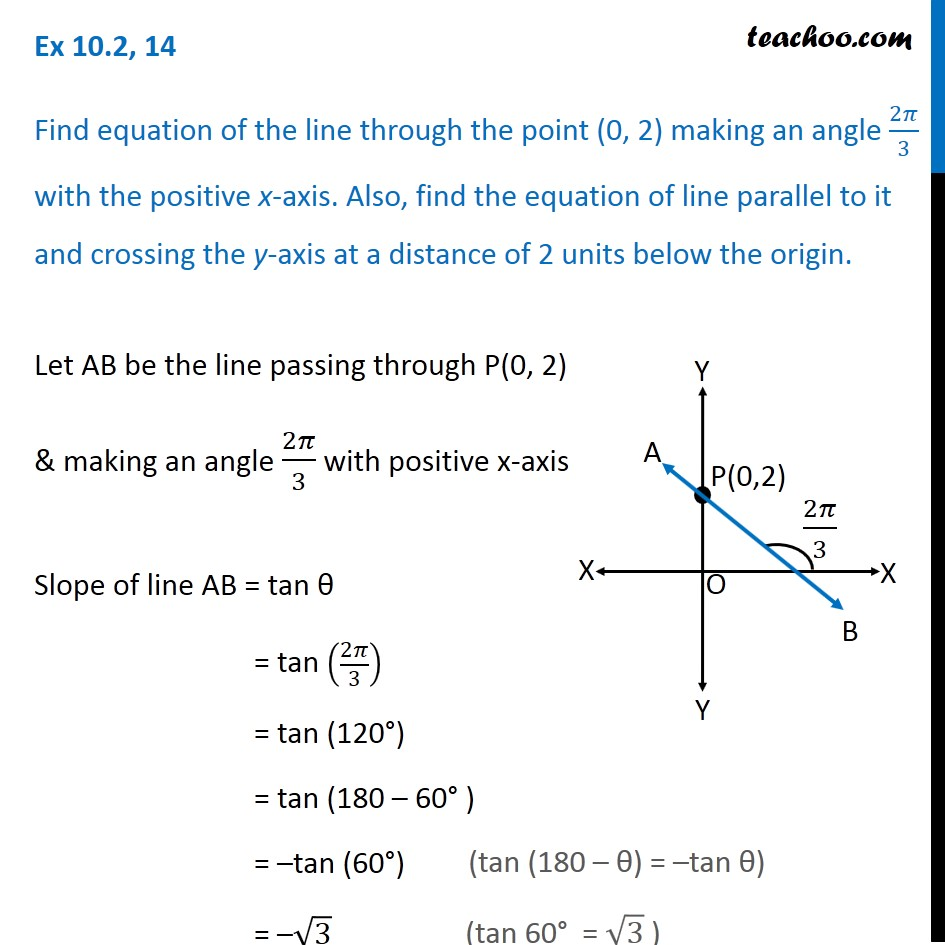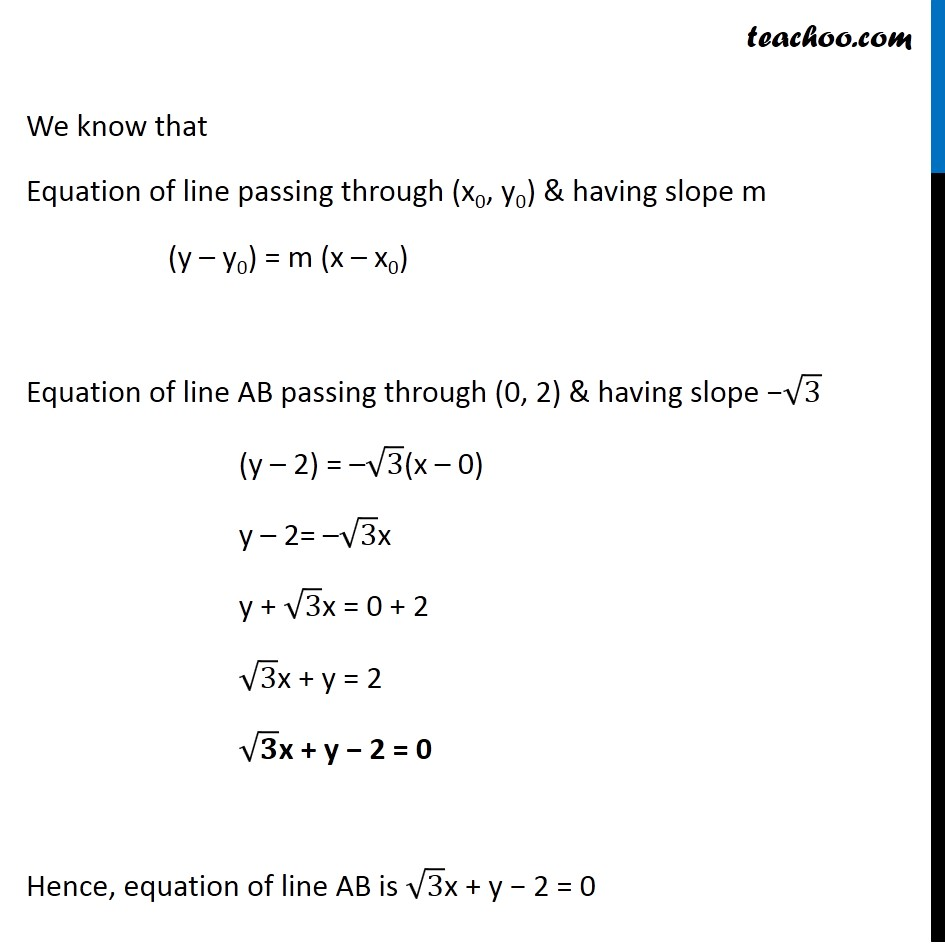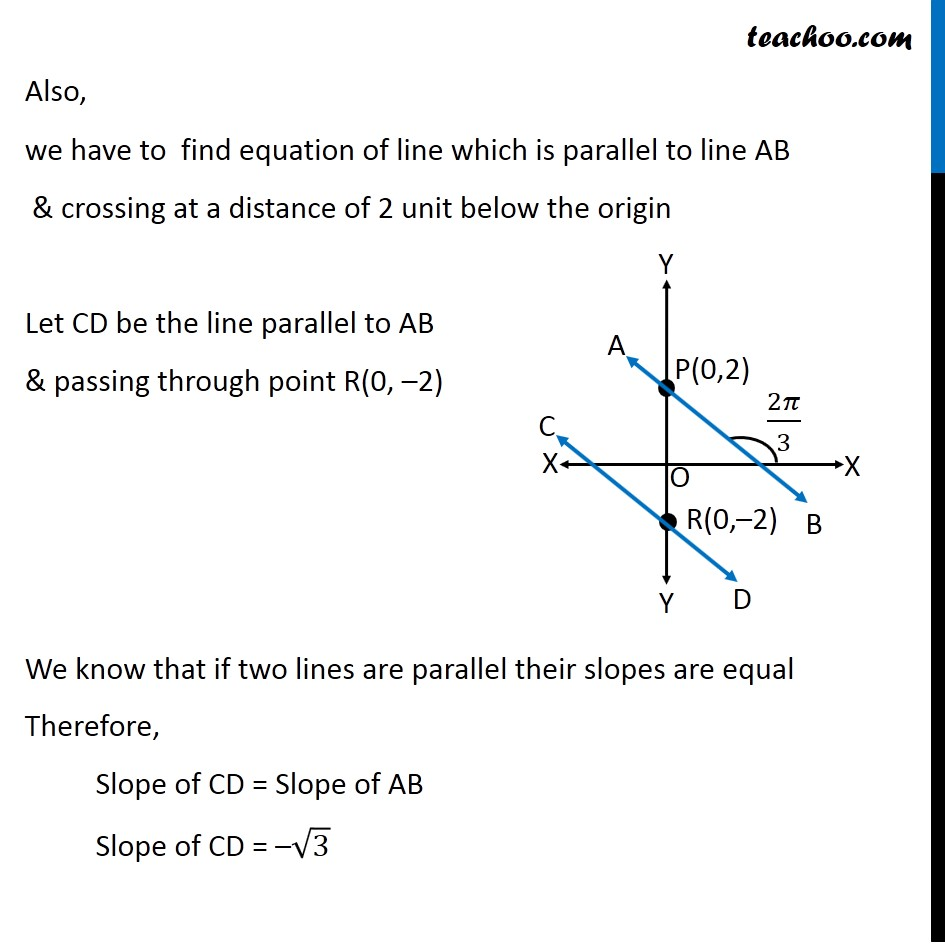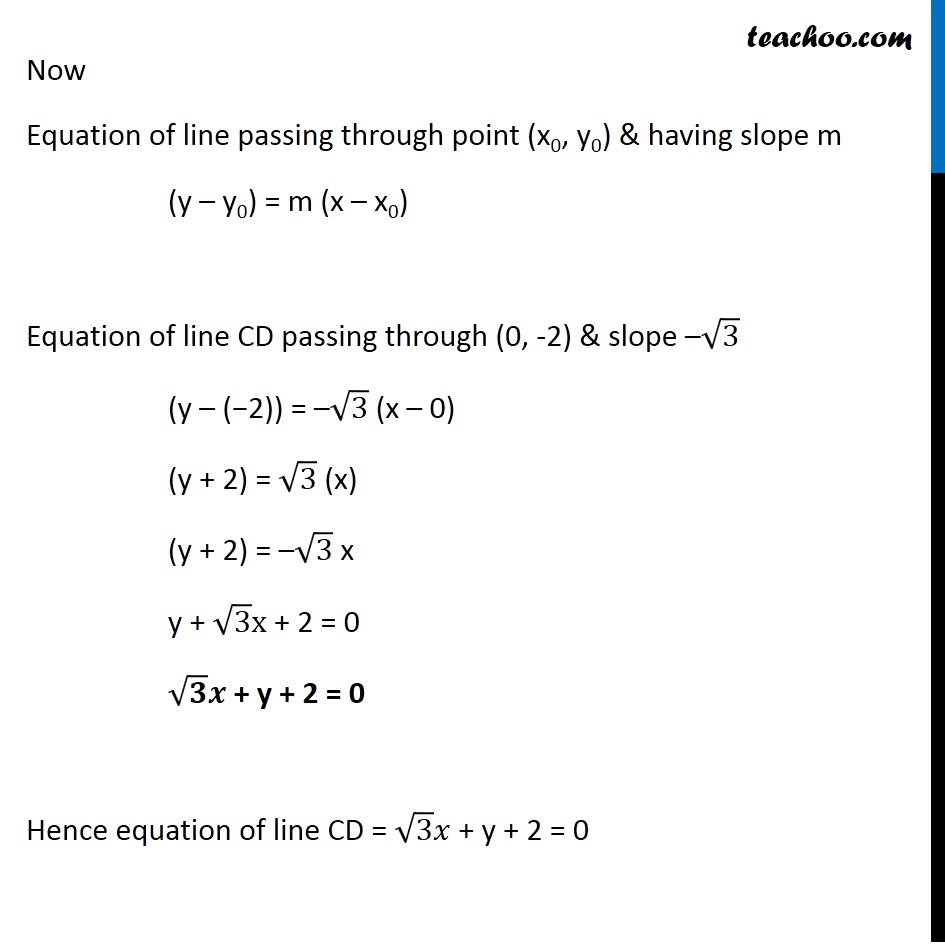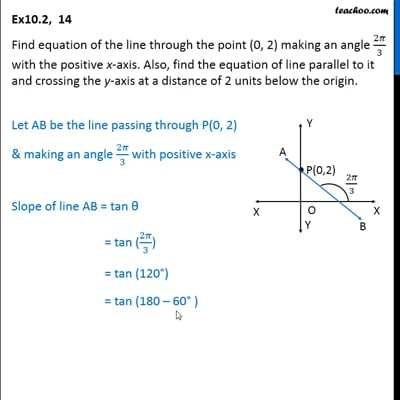This video is only available for Teachoo black users

### Transcript

Ex 10.2, 14 Find equation of the line through the point (0, 2) making an angle 2𝜋/3 with the positive x-axis. Also, find the equation of line parallel to it and crossing the y-axis at a distance of 2 units below the origin. Let AB be the line passing through P(0, 2) & making an angle 2𝜋/3 with positive x-axis Slope of line AB = tan θ = tan (2𝜋/3) = tan (120°) = tan (180 – 60° ) = –tan (60°) = –√3 (tan (180 – θ) = –tan θ) (tan 60° = √3 ) We know that Equation of line passing through (x0, y0) & having slope m (y – y0) = m (x – x0) Equation of line AB passing through (0, 2) & having slope −√3 (y – 2) = –√3(x – 0) y – 2= –√3x y + √3x = 0 + 2 √3x + y = 2 √𝟑x + y − 2 = 0 Hence, equation of line AB is √3x + y − 2 = 0 Also, we have to find equation of line which is parallel to line AB & crossing at a distance of 2 unit below the origin Let CD be the line parallel to AB & passing through point R(0, –2) We know that if two lines are parallel their slopes are equal Therefore, Slope of CD = Slope of AB Slope of CD = –√3 Now Equation of line passing through point (x0, y0) & having slope m (y – y0) = m (x – x0) Equation of line CD passing through (0, -2) & slope –√3 (y – (−2)) = –√3 (x – 0) (y + 2) = √3 (x) (y + 2) = –√3 x y + √3 x + 2 = 0 √𝟑 𝒙 + y + 2 = 0 Hence equation of line CD = √3 𝑥 + y + 2 = 0